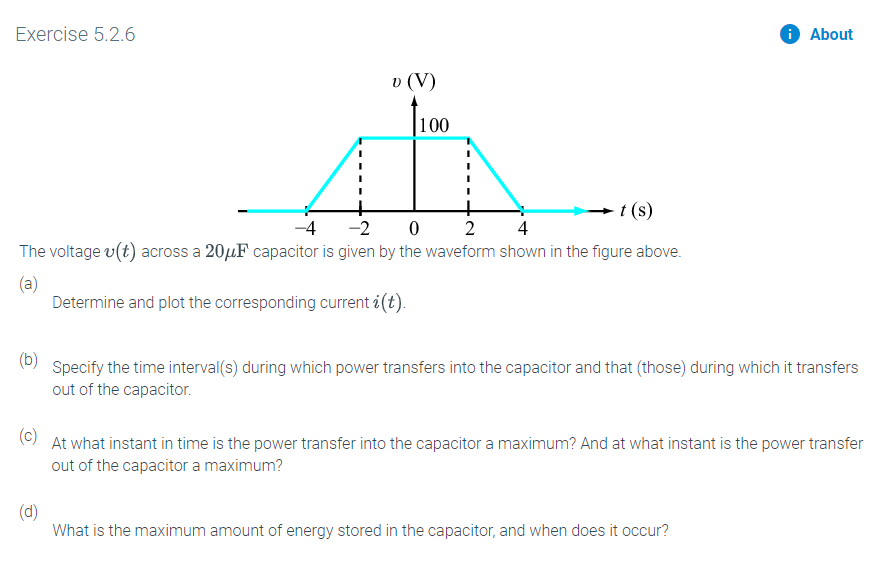# Exercise 5.2.6AboutL00t (s)-2-4024The voltage v(t) across a 20HF capacitor is given by the waveform shown in the figure above.(a)Determine and plot the corresponding current i(t)(b)Specify the time interval(s) during which power transfers into the capacitor and that (those) during which it transfersout of the capacitor.(c)At what instant in time is the power transfer into the capacitor a maximum? And at what instant is the power transferout of the capacitor a maximum?(d)What is the maximum amount of energy stored in the capacitor, and when does it occur?

Question
19 viewshelp_outlineImage TranscriptioncloseExercise 5.2.6 About L00 t (s) -2 -4 0 2 4 The voltage v(t) across a 20HF capacitor is given by the waveform shown in the figure above. (a) Determine and plot the corresponding current i(t) (b) Specify the time interval(s) during which power transfers into the capacitor and that (those) during which it transfers out of the capacitor. (c) At what instant in time is the power transfer into the capacitor a maximum? And at what instant is the power transfer out of the capacitor a maximum? (d) What is the maximum amount of energy stored in the capacitor, and when does it occur? fullscreen
check_circle

star
star
star
star
star
1 Rating
Step 1

The maximum energy stored in the capacitor, the time interval of power transferred to the capacitor, power out from the capacitor, maximum power to the capacitor and out of the capacitor has to be determined when following voltage waveform is applied to the capacitor with C = 20 µF

Step 2

Current waveform from part (a):

Step 3

The power can be calculated be the formula:

P(t) = v(t)×i(...

### Want to see the full answer?

See Solution

#### Want to see this answer and more?

Solutions are written by subject experts who are available 24/7. Questions are typically answered within 1 hour.*

See Solution
*Response times may vary by subject and question.
Tagged in

### Electrical Engineering